Courses
Courses for Kids
Free study material
Offline Centres
More

# Drawing Parallel Lines with Set SquareLast updated date: 02nd Dec 2023
Total views: 101.1k
Views today: 3.01k## What is Set Square

A set square or triangle is an object used in engineering and technical drawing, with the aim of providing a straightedge at a right angle or another particular planar angle to a baseline.

The simplest form of a set square is a triangular piece of transparent plastic (or formerly of polished wood) with the center removed. More commonly the set square bears the markings of a ruler and a half-circle protractor.

Using a fixed square to draw parallel lines is an accurate method. A rectangular drawing tool called a "set square" is used to draw vertical lines, parallel lines, and some common angles. Lines that never cross each other are said to be parallel.

## Parallel Lines

Two lines in the same plane that are equally spaced apart and never cross each other are said to be parallel lines in geometry. Both horizontal and vertical shapes are possible. Examples of parallel lines can be found everywhere, including zebra crossings, rows of notebooks, and the nearby railroad tracks.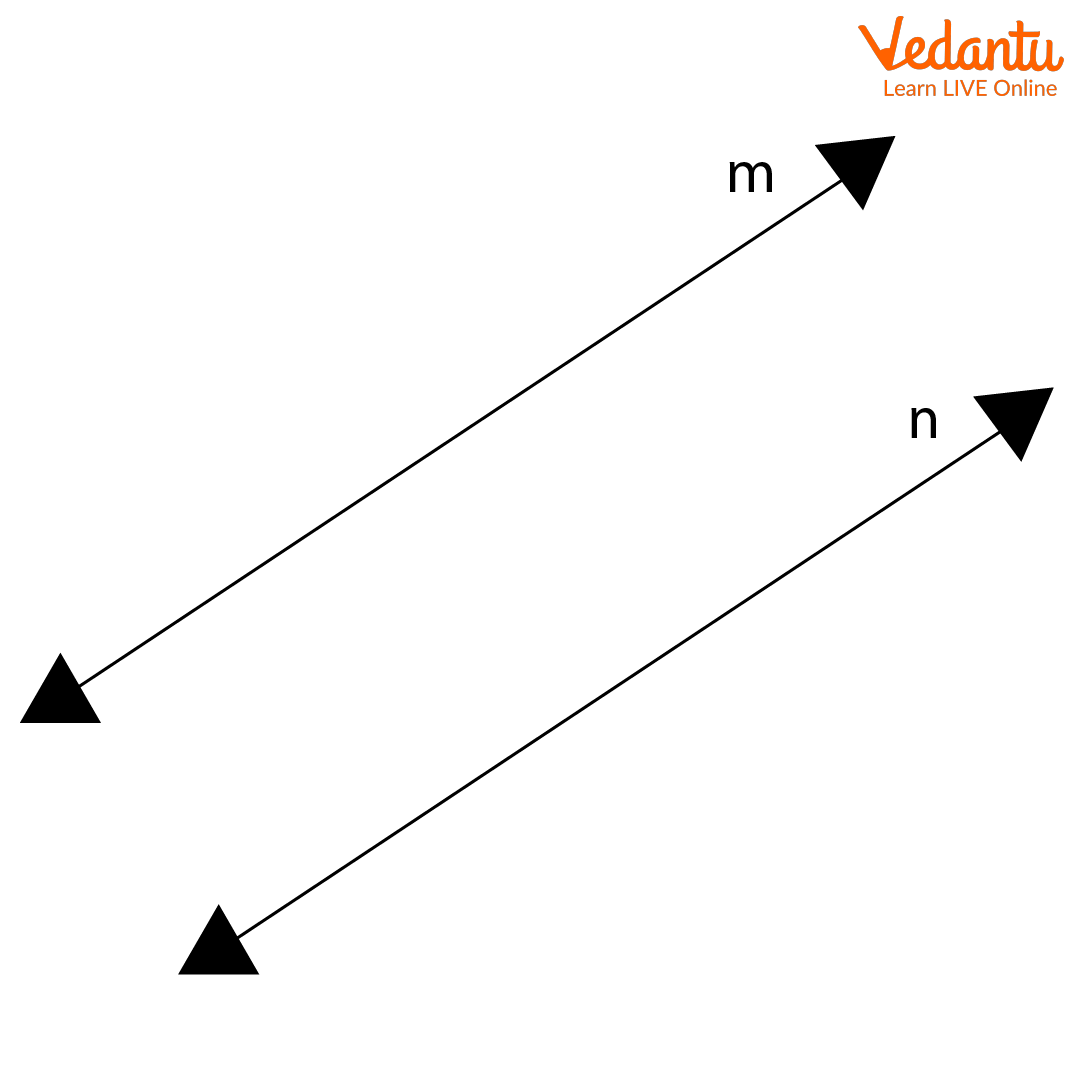Parallel Lines

## What is Set Square

A set square is used in technical drawings and math practice to represent predefined angles. Setting rectangles are typically made of thin plastic, wood, or, in rare cases, thin steel and have a triangular shape. Every plane represents three different angles, always containing one right angle. There are two common combinations of given set square angles: the ${30^{^o}} - {60^o} - {90^o}$ option and the ${45^o} - {45^o} - {90^o}$ option.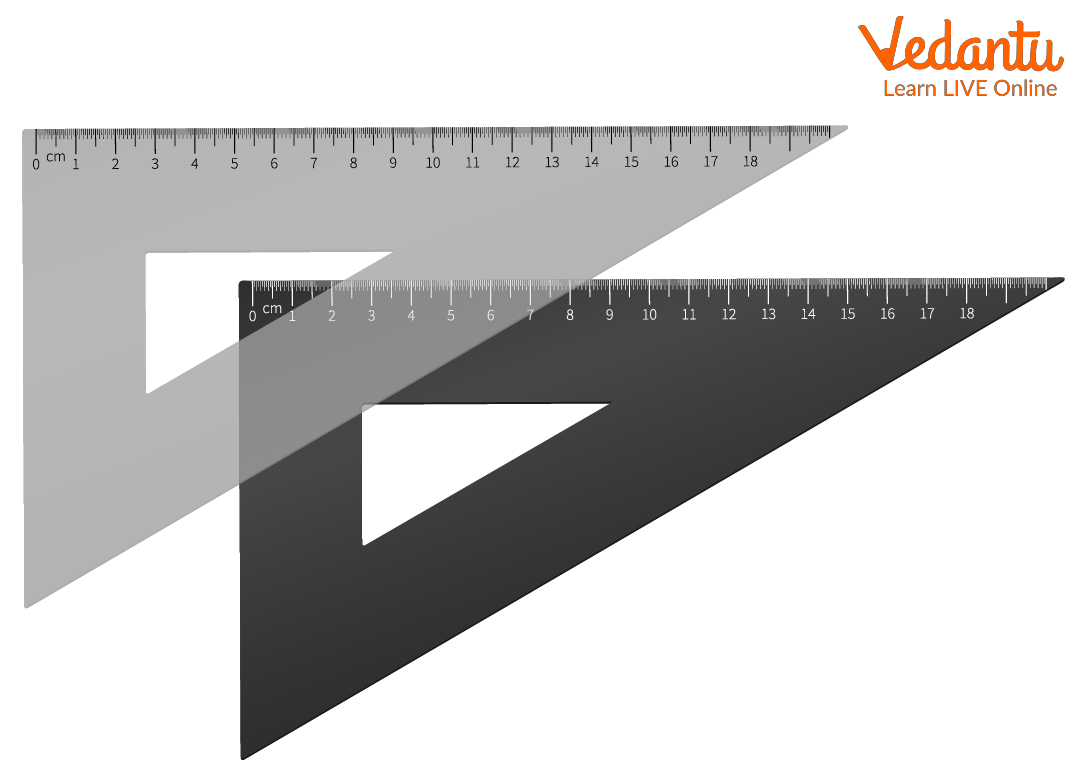Set Square

## Correct Use of Set Square

To use set squares correctly:

• One side of the desired angle set must be set on a solid, level bottom line, such as the cross-arm of a T-square that is typically perpendicular to the paper and is oriented at a known angle.

• The other edge of the established angle is then often marked off using a pencil or pen.

## Use of Set Square

Following is the list of the uses of set square:

• Draw a vertical line

• Draw vertical corners

• Draw a standard measurement angle [the two given rectangles are named after their angles; one is 90, 30, and 60 degrees, and the other is 90 and 45 degrees.

• Parallel lines can be drawn with the help of set square

## Drawing Parallel Lines Using the Set Square

1. Draw a line l and mark a point outside the line.

2. Place any side of the set square forming the right angle along line l.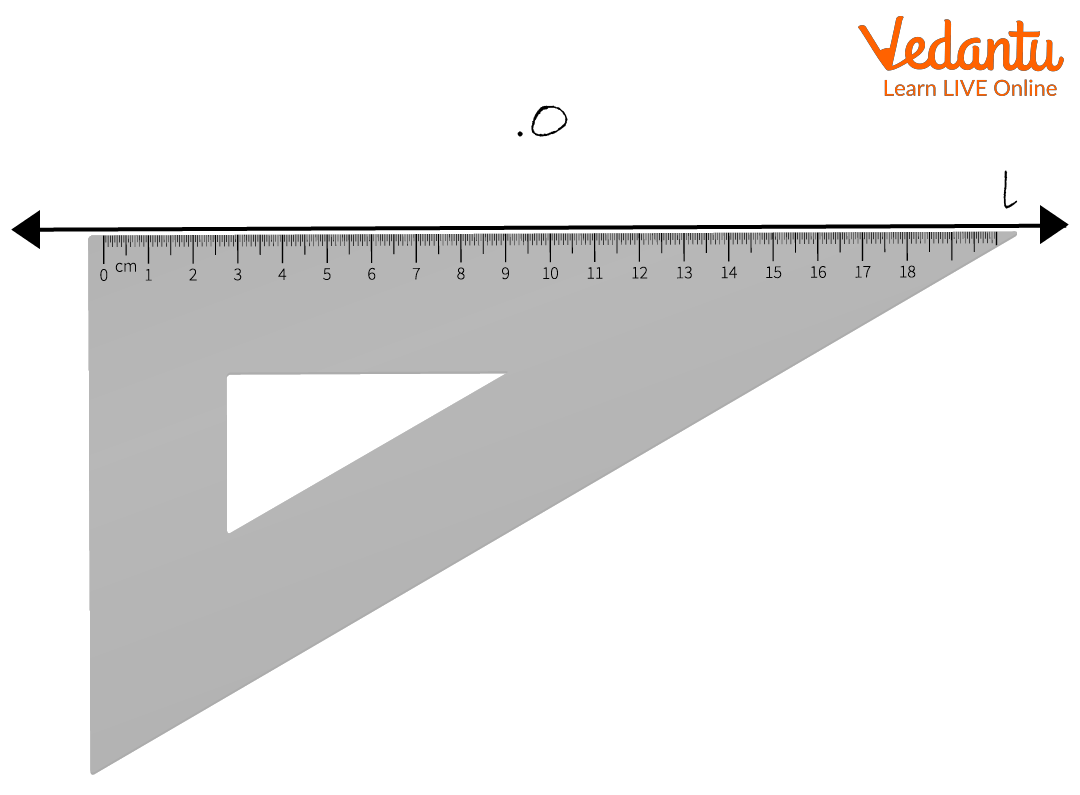Drawing a Line

3. Place a ruler along the other side of the set square to form a right angle as shown.

The line must be fixed.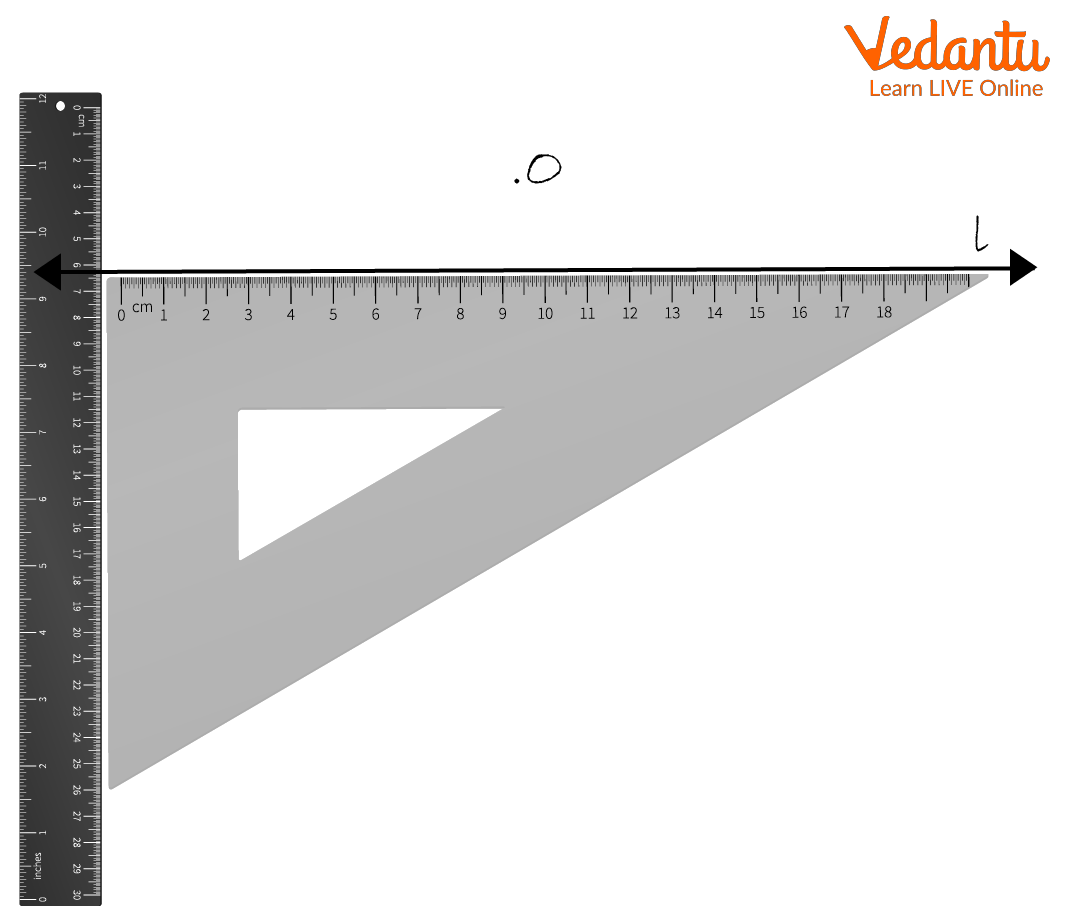Place a Ruler Alongside

4. Slide the set square along the ruler upwards such that point O lines along the arm of the set square.

5. Remove the ruler and draw a line along the given line. Name this line m.

m is the desired line parallel to l. m|| l.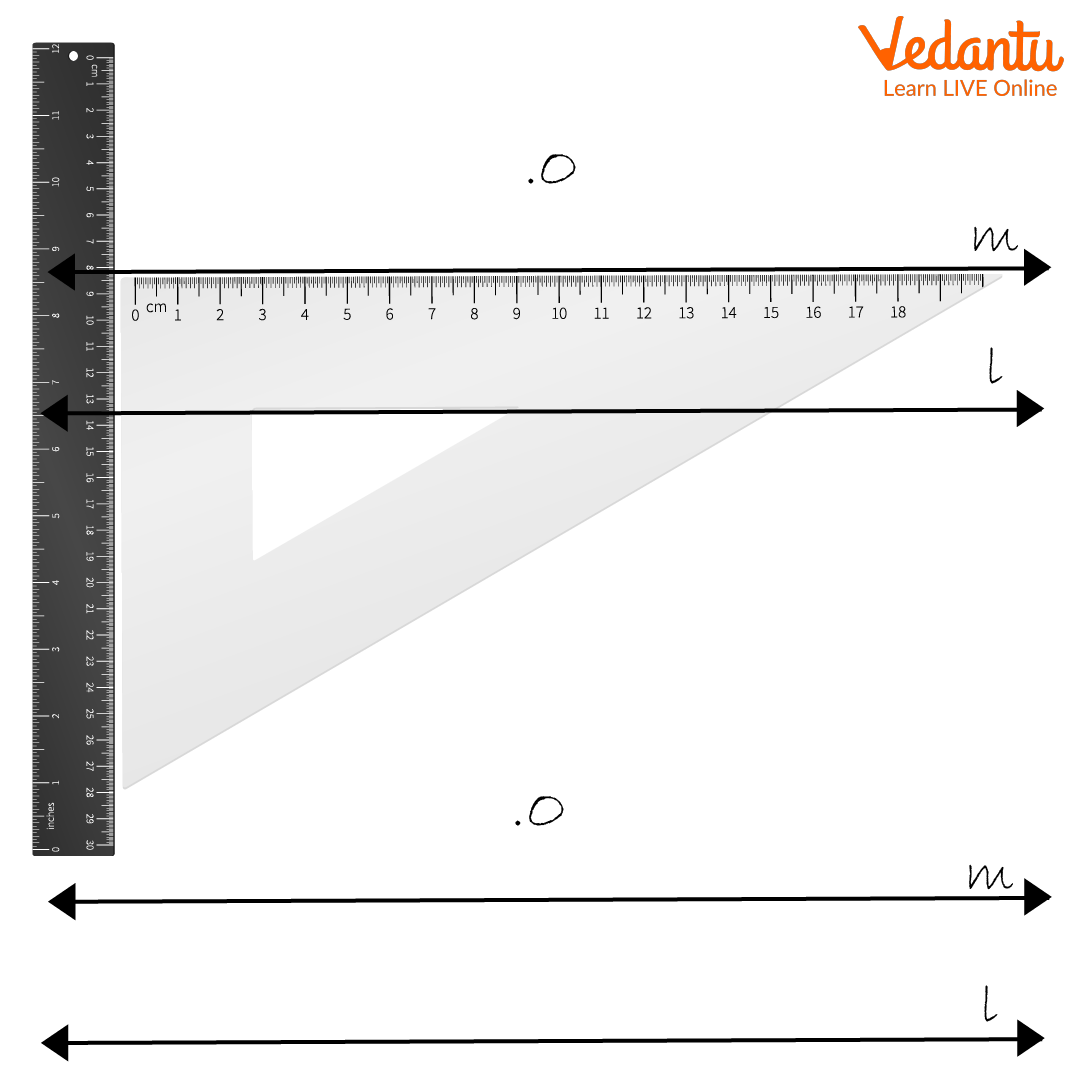Drawing Parallel Lines

## Solved Examples

Using a square and ruler, draw a line parallel to this line way through the 5cm point above.

(i) Use a ruler to draw an ${\rm{XY}}$ line and mark point A on it.

(ii) Draw ${\rm{AM}} = 5$cm using a square.

(iii) Place the given square on the ${\rm{XY}}$ segment.

(iv) While holding the straightedge firmly along ${\rm{AM}}$ move the rectangle along the straightedge until the edge of the rectangle touches point M

(v) Draw a line ${\rm{MN}}$ along the edge through M.

(vi) ${\rm{MN}}$ is the desired line from M parallel to ${\rm{XY}}$.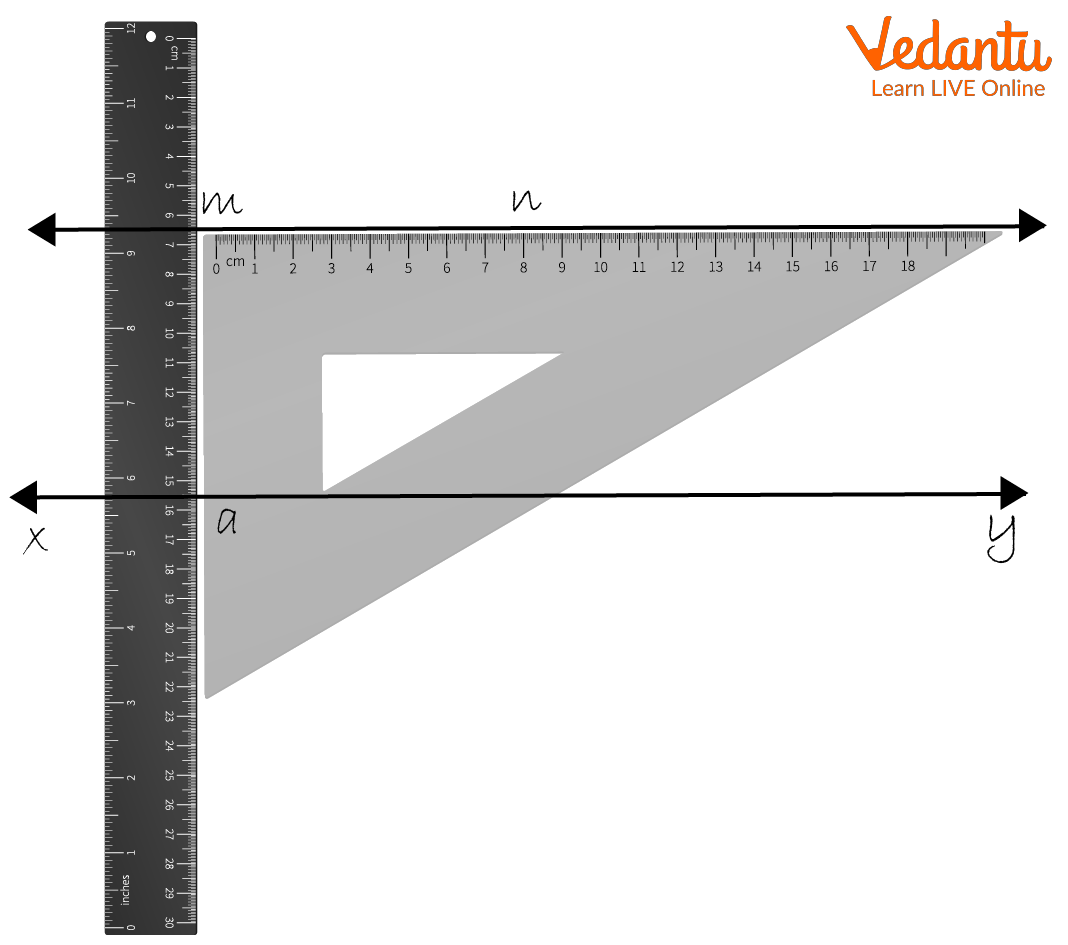Drawing Parallel Lines

## Conclusion

The cross-arm of a T-square, which is typically perpendicular to the paper and is oriented at a specified angle, or another solid, level bottom line, must be placed on one side of the desired angle. Then, it's common practice to mark off with a pencil or pen the other edge of the agreed angle.

## FAQs on Drawing Parallel Lines with Set Square

1. Write some properties of parallel lines.

• Two or more lines that lie in the same plane and never intersect are called parallel lines.

• Parallel lines are straight lines that never intersect no matter how much you stretch them.

• The symbol used to indicate parallel lines is ॥.

• Parallel lines are always straight lines that are the same distance apart.

• Parallel lines never intersect no matter what direction they extend.

2. What is the proper way to use set squares?

They need to have one side of the desired angle set placed on a stable, flat bottom line, like the cross-arm of a T-square orientated at a known angle typically perpendicular to the paper. A pencil or pen is then wont to mark off the angle along the other edge of the angle set.

3. Write the applications of the set square.

Set square can be used for:

1. Drawing perpendicular lines

2. Draw parallel edges

3. Draw some standard measured angles( The two set squares are named according to their angles. One has 90, 30, and 60-degree angles and the other has 90 and 45-degree angles.)

4. Can be real good ruler when you forget to bring it with you